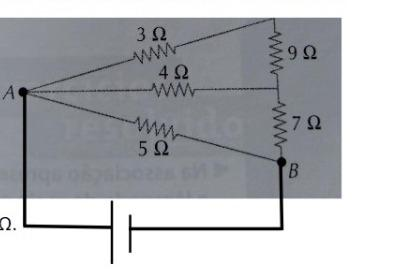# Question In the association of resistors on the side, an ideal voltage source of 10 V has been connected between points A and B. Determine: (a) The equivalent resistance of the circuit. (b) The total electrical current in the circuit. (c) The potential difference across the 7 ohm resistor terminals. (d) The electric current through the 4 ohm resistor. 3 Ω ΑΛΜ 9Ω 4 Ω ΑΛΛΜ A 7 Ω 5Ω B 2.GXYOGQ The Asker · Electrical EngineeringIn the association of resistors on the side,
an ideal voltage source of 10 V has been connected
between points A and B. Determine:

(a) The equivalent resistance of the circuit.
(b) The total electrical current in the circuit.
(c) The potential difference across the 7 ohm resistor terminals.
(d) The electric current through the 4 ohm resistor.

Transcribed Image Text: 3 Ω ΑΛΜ 9Ω 4 Ω ΑΛΛΜ A 7 Ω 5Ω B 2.
More
Transcribed Image Text: 3 Ω ΑΛΜ 9Ω 4 Ω ΑΛΛΜ A 7 Ω 5Ω B 2.